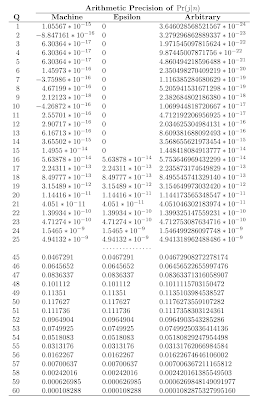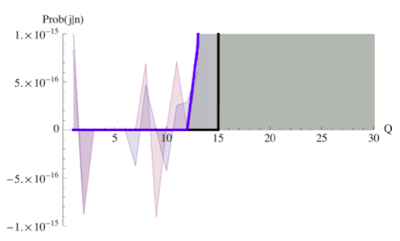## Monday, January 14, 2008

### What to Do with Wobbly Numbers in PDQ

Peter Altvogt (Germany) reported an interesting numerical effect while using the load-dependent server model in Section 6.7.11 of "Analyzing Computer System Performance with Perl::PDQ". The default parameters in fesc.pl are set to N = 15 users and M = 3 maximum processes allowed in the sub-system. The PDQ model calculates the joint probability distribution Pr(j|n) of the queue length at the load-dependent server. For these parameters the mean queue length is 4.50 users.

The effect that Peter observed can be replicated by choosing N = 60 while keeping M = 3. A plot of the corresponding Pr(j|n) shows that the mean queue length (the peak location) moves to 48.42 users.However, at some points in the calculation of Pr(j|n) by:

$pq[$n] -= $pq[$j][$n]; this choice of parameters introduces a determination of the difference between two very similar and very small numbers.As a consequence, the limitations of the floating-point representations begin to show up as tiny negative values for the probabilities, which are disallowed by definition. NOTE: This effect is not caused by PDQ. Rather, it is a classic case of the type discussed in "Numerical Recipes". The problem has to do with the potential limitations due to the choice of finite internal representation of numbers in general and non-exact numbers in floating-point format, in particular. The table at left (click on it to get a bigger image) shows explicitly how the degree of error can be traded off against computational time. The left column shows the original effect; negative values of the probability near zero queue length when calculated with fast machine-precision FP. The middle column shows what happens if a numerical cut-off condition, like the one discussed in "Mastering Algorithms with Perl", is introduced. Peter implemented a cut-off for fesc.pl as: $eps = 1.0e-10;
...
$pq[$n] -= $pq[$j][$n]; if ( abs($pq[$n]) <$eps ) {
$pq[$n] = 0.0;
}

and this is probably the most expeditious method for PDQ models (in any language). In the above table, I reproduced the same approach using the Mathematica parameter:
100 * \$MachineEpsilon
which is around 10-14 on my PowerPC. As you can see, the cut-off replaces the original offending negative probabilities with zeros. A less artificial result can also be achieved in Mathematica because it has the capability of doing arithmetic with arbitrary precision numbers (in software). Those results are shown in the right column of the table. The values that were previously negative or cut off at zero, are now shown as extremely tiny numbers with precision beyond that which can be represented in hardware. A combined plot summarizes it best.At the level of machine precision (the fastest), FP numbers can go wobbly on you. The black line shows the effect of introducing a cut-off, which sharply jumps up from zero (hence the name). The blue line shows the more graceful, and more computationally intensive, effect of calculating with arbitrary precision arithmetic. Note carefully the magnitudes on the y-axis. There is no easy way around this effect, so one has to remain vigilant with all computations, no matter how they are done. Even standards like IEEE FP 754 cannot prevent these problems. At least in this case, the negative probabilities provided a tip-off.